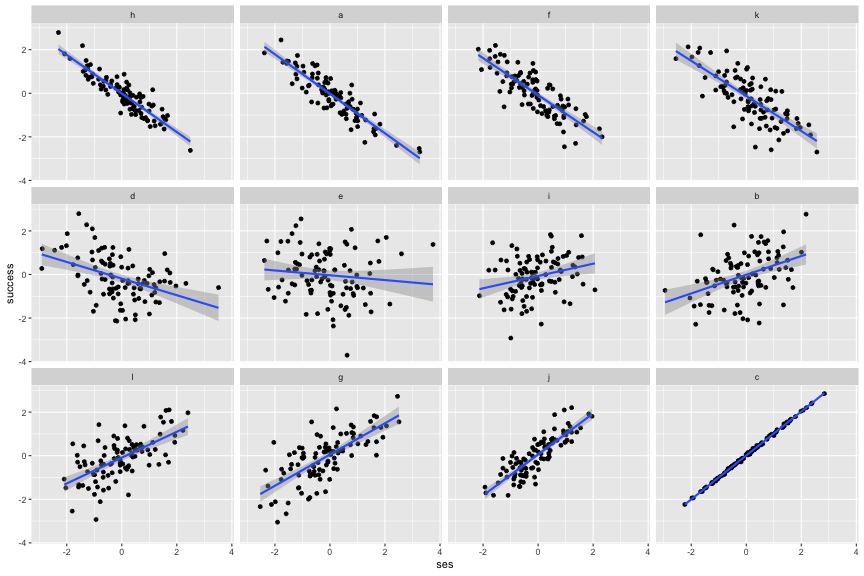## Introduction

This post will show you how to order small multiples in ggplot2 by arbitrary criteria. It is straightforward to generate small multiples in ggplot2: Add either `facet_grid()` or `facet_wrap()` to your code and set its first argument to (preferably) a set of factors which defines faceting groups. The order of the resulting small multiples will follow the order of the provided factor levels. In other words, if you want to sort small multiples by arbitrary criteria, the you will have to reorder the underlying factor levels.

## Motivation

Assume you have data, measuring the impact of socioeconomic status on student success. Students are nested in school districts. You want to: (1) Plot the relationship for each school district; (2) Order districts by the magnitude of the correlation (such that you might hypothesize possible similiarities between districts). Here is what your toy data look like:

ses success district_id
1196 -1.6179474 0.0188662 l
572 0.0252540 -0.3111760 f
138 -0.4647769 -0.5836298 b
883 1.5759108 1.7807955 i
1168 0.2507581 -0.4603069 l
610 1.9244564 0.8464252 g
1141 -0.8316925 1.4239423 l
1074 0.8881493 0.1626624 k
988 -1.4506733 -1.2485325 j
1148 -0.1606711 0.2567397 l

## Recipe

The variable district_id identifies each school district and, consequently, the small multiples you are after. The order of its levels should match the magnitude of the within-district correlation between variables ses and success. We need to (1) compute said correlation, (2) reorder district levels by its value, and (3) plot the data.

``````# Step (1): Calculate the within district correlation
r_district <- vector("numeric", length(unique(ses_data[["district_id"]])))
names(r_district) <-  unique(ses_data[["district_id"]])
for(i in names(r_district)){
filter <- which(ses_data\$district == i)
r_district[i] <- cor(ses_data[filter, "ses"], ses_data[filter, "success"])
}

# Step (2): Reorder the factor levels
levels(ses_data\$district_id) # before
``````
```##   "a" "b" "c" "d" "e" "f" "g" "h" "i" "j" "k" "l"
```
``````ses_data <- within(ses_data,
district_id <- factor(district_id, names(r_district)[order(r_district)])
)
levels(ses_data\$district_id) # after
``````
```##   "h" "a" "f" "k" "d" "e" "i" "b" "l" "g" "j" "c"
```
``````# Step (3): Plot the data
library("ggplot2")
ggplot(data = ses_data, aes(x = ses, y = success)) +
geom_point() + geom_smooth(method = "lm") +
facet_wrap(vars(district_id))
``````## Conclusion

In this post you have seen how small multiples in ggplot2 can be sorted by arbitrary criteria. Although the toy example is limited to the case of `facet_wrap()` and a single grouping factor, the approach generalizes. No matter what wrapping function or how many facets you define: The order of small multiple plots follows the order of the underlying factor levels.

## Toy Data Creation

``````# Generate toy data with multilevel structure
rm(list = ls())

# Set population level parameters
n_districts <- 12 # number of school districts
n_students <- 100 # number of students per district
rho <- .4 # mean effect of spending

# Draw district level correlation r
r <- rnorm(n_districts, psych::fisherz(rho), sd = 1.5)
r <- psych::fisherz2r(r)

# Draw district level data
ses_data <- data.frame()
for(i in r){
Sigma <- matrix(c(1, i, i, 1), 2, 2)
ses_data <- rbind.data.frame(ses_data, MASS::mvrnorm(n_students, c(0, 0), Sigma))
}
rm(i, Sigma)
names(ses_data) <- c("ses", "success")
ses_data[, "district_id"] <- factor(
rep(letters[seq(n_districts)], each = n_students)
)
## END
``````